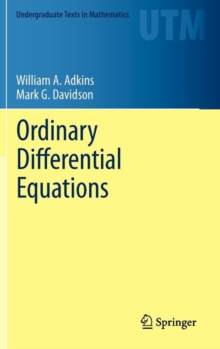Supporting your high street Find out how »
• My Account# Ordinary Differential Equations Hardback

## Part of the Undergraduate Texts in Mathematics series

#### Description

Unlike most texts in differential equations, this textbook gives an early presentation of the Laplace transform, which is then used to motivate and develop many of the remaining differential equation concepts for which it is particularly well suited.

For example, the standard solution methods for constant coefficient linear differential equations are immediate and simplified, and solution methods for constant coefficient systems are streamlined.

By introducing the Laplace transform early in the text, students become proficient in its use while at the same time learning the standard topics in differential equations.

The text also includes proofs of several important theorems that are not usually given in introductory texts.

These include a proof of the injectivity of the Laplace transform and a proof of the existence and uniqueness theorem for linear constant coefficient differential equations. Along with its unique traits, this text contains all the topics needed for a standard three- or four-hour, sophomore-level differential equations course for students majoring in science or engineering.

These topics include: first order differential equations, general linear differential equations with constant coefficients, second order linear differential equations with variable coefficients, power series methods, and linear systems of differential equations.

It is assumed that the reader has had the equivalent of a one-year course in college calculus.

#### Information

• Format: Hardback
• Pages: 799 pages, 28 Tables, black and white; 121 Illustrations, black and white; XIII, 799 p. 121 illus.
• Publisher: Springer-Verlag New York Inc.
• Publication Date:
• Category: Differential calculus & equations
• ISBN: 9781461436171

#### Other Formats

£53.99

£45.05

on all orders

###### Pick up orders

from local bookshops

£64.99

£55.24

£53.99

£45.89

£53.99

£45.05

£44.99

£37.35

£46.99

£38.75

£34.99

£30.09

£44.99

£36.95